# 卡尔曼滤波器原理说明

1.通过观测一个运动系统的输入输出，综合考虑运动误差（假定是高斯分布）和观测误差（假定为高斯分布），来预测系统的真实状态：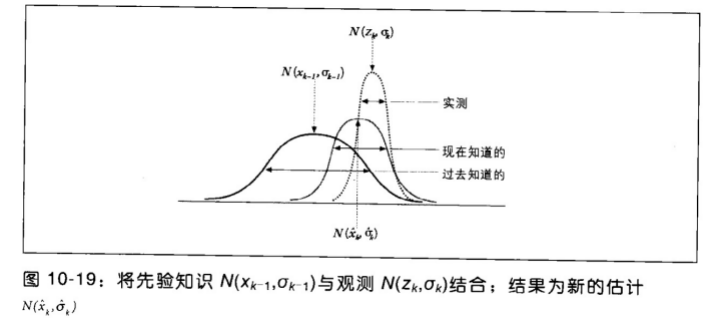'A:',kalman.transitionMatrix（转化矩阵，将当前时刻的Xk转化为下一时刻的Xk+1，初始化后不变）
'H:',kalman.measurementMatrix（观测矩阵，将系统状态X转化为观测值的矩阵，初始化后不变）
'Q:',kalman.processNoiseCov（过程误差协方差矩阵，表示在系统运行过程时的误差程度，值越高表示误差越大，初始化后不变）
'R:',kalman.measurementNoiseCov（观测误差协方差矩阵，表示观测值的误差程度，值越高表示越不信任观测值，初始化后不变）
'P:',kalman.errorCovPost（状态协方差矩阵，用于描述系统状态中各个变量的相关性，根据系统状态不断更新）
'X:',kalman.statePost（状态矩阵，根据系统状态不断更新）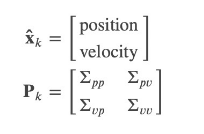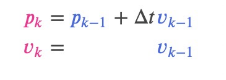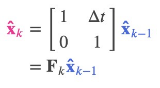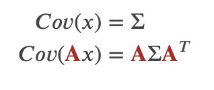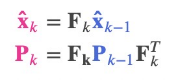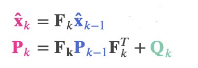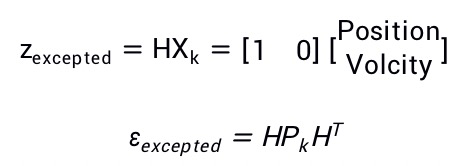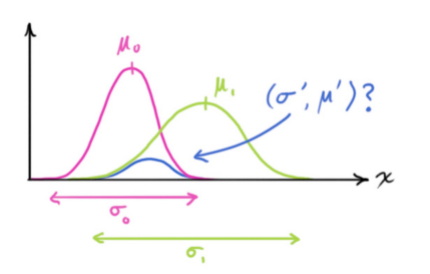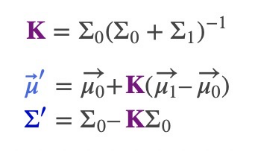void KalmanFilter::init(int DP, int MP, int CP, int type)
{
statePre = Mat::zeros(DP, 1, type);   //预测状态
statePost = Mat::zeros(DP, 1, type);   //当前状态X
transitionMatrix = Mat::eye(DP, DP, type);   //转化矩阵A
processNoiseCov = Mat::eye(DP, DP, type);   //过程误差矩阵Q
measurementMatrix = Mat::zeros(MP, DP, type);  //观测转化矩阵H
measurementNoiseCov = Mat::eye(MP, MP, type);  //观测误差协方差矩阵R
errorCovPre = Mat::zeros(DP, DP, type);   //预测状态协方差矩阵
errorCovPost = Mat::zeros(DP, DP, type);  //当前状态协方差矩阵P
gain = Mat::zeros(DP, MP, type);  //卡尔曼增益K，计算时自动生成
if (CP > 0)
controlMatrix = Mat::zeros(DP, CP, type);  //控制变量矩阵，暂用不到
else
controlMatrix.release();
temp1.create(DP, DP, type);
temp2.create(MP, DP, type);
temp3.create(MP, MP, type);
temp4.create(MP, DP, type);
temp5.create(MP, 1, type);
}

const Mat &KalmanFilter::predict(const Mat &control)
{
// update the state: x'(k) = A*x(k)
statePre = transitionMatrix * statePost;

if (!control.empty())
// x'(k) = x'(k) + B*u(k)
statePre += controlMatrix * control;

// update error covariance matrices: temp1 = A*P(k)
temp1 = transitionMatrix * errorCovPost;

// P'(k) = temp1*At + Q
gemm(temp1, transitionMatrix, 1, processNoiseCov, 1, errorCovPre, GEMM_2_T);

// handle the case when there will be measurement before the next predict.
statePre.copyTo(statePost);
errorCovPre.copyTo(errorCovPost);

return statePre;
}

const Mat &KalmanFilter::correct(const Mat &measurement)
{
// temp2 = H*P'(k)
temp2 = measurementMatrix * errorCovPre;
// temp3 = temp2*Ht + R
gemm(temp2, measurementMatrix, 1, measurementNoiseCov, 1, temp3, GEMM_2_T);
// temp4 = inv(temp3)*temp2 = Kt(k)
solve(temp3, temp2, temp4, DECOMP_SVD);
// K(k)
gain = temp4.t();
// temp5 = z(k) - H*x'(k)
temp5 = measurement - measurementMatrix * statePre;
// x(k) = x'(k) + K(k)*temp5
statePost = statePre + gain * temp5;
// P(k) = P'(k) - K(k)*temp2
errorCovPost = errorCovPre - gain * temp2;

return statePost;
}

04-292427
12-31199709-033298
03-244771
01-182万+
04-29365
01-112650
02-127823